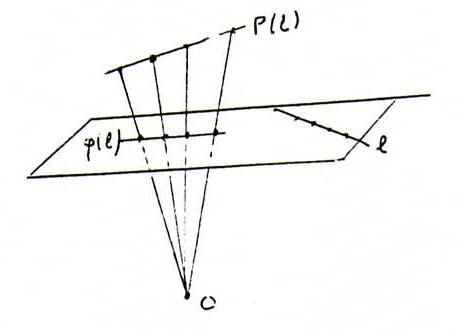### COURSE OF PROJECTIVE GEOMETRY

§ 15:

O59 Let A, B, C, D be the freely situated fixed points.So the intersection point S1 of AB and CD is a fixed point.
Then both of the lines AB and CD contain three fixed points, so they are pointwise invariant (FS §10). Likewise, each of the lines of the complete quadrangle ABCD is pointwise invariant.
Now let X be an arbitrary point in P2. Choose a line through X that intersects the complete quadrangle ABCD in at least three points. Then this line contains three fixed points, so it is pointwise invariant. So X is a fixed pont. Since this holds for each X, the projective mapping is identity.

O60 That φ induces a bijection θ from the pencil of lines L to the pencil of lines φ(L) follows from the definition of a collineation. Because φ is a projective transformation, θ preserves cross ratio. According to the second proposition of §10, θ is a projectivity.
If φ leaves the two pencils linewise invariant,we easily find four freely situated fixed points,because the intersection point of two invariant lines is a fixed point. According to O59, φ is identity.

O61 Let c = a + λ(b - a), with λ outside {0,1}. Then P(c) = P(a) + λ(P(b) - P(a)).
So P(a), P(b), P(c) are collinear and P(A)P(B) / P(A)P(C) = ||P(a) - P(b)|| / ||P(a) - P(c)|| = 1/|λ| = ||a - b|| / ||a - c|| = AB / AC.
Now if A, B, C, D are four points on a line l in {x3 = 1}, we find:
(A, B; C, D) = (P(A), P(B); P(C), P(D)) = (φ(A), φ(B); φ(C), φ(D)) (projection of P(l) onto φ(l) from O).